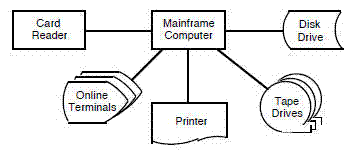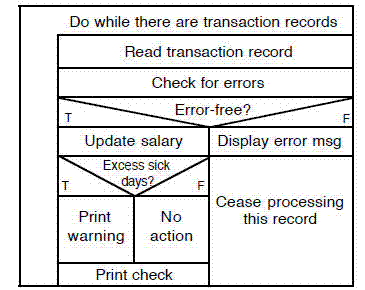## Search Results

### 5 results were found.

Print Results
 block diagram. (1) diagram of a system in which the principal parts or functions are represented by blocks connected by lines that show the relationships of the blocks (2) diagram of a system, computer, or device in which the principal parts are represented by suitably annotated geometrical figures to show both the functions of the parts and their functional relationships Syn: configuration diagram, system resources chart See Also: box diagram, bubble chart, flowchart, graph, input-process-output chart, structure chartbox diagram. (1) control flow diagram consisting of a rectangle that is subdivided to show sequential steps, if-then-else conditions, repetition, and case conditions Syn: Chapin chart, Nassi-Shneiderman chart See Also: block diagram, bubble chart, flowchart, graph, input-process-output chart, program structure diagram, structure chartbubble chart. (1) data flow, data structure, or other diagram in which entities are depicted with circles (bubbles) and relationships are represented by links drawn between the circles See Also: block diagram, box diagram, flowchart, graph, input-process-output chart, structure chartinput-process-output (IPO) chart. (1) diagram of a software system or module, consisting of a rectangle on the left listing inputs, a rectangle in the center listing processing steps, a rectangle on the right listing outputs, and arrows connecting inputs to processing steps and processing steps to outputs Note: summarizes the process activities and their inputs and outputs from/to external actors; some inputs are categorized as controls and enablers. Syn: IPO diagram See Also: block diagram, box diagram, bubble chart, flowchart, graph, structure chartflowchart. (1) graphical representation of a process or the step-by-step solution of a problem, using suitably annotated geometric figures connected by flowlines for the purpose of designing or documenting a process or program (2) graphical representation of the definition, analysis, or method of solution of a problem in which symbols are used to represent operations, data, flow, equipment, etc (3) control flow diagram in which suitably annotated geometrical figures are used to represent operations, data, or equipment, and arrows are used to indicate the sequential flow from one to another (4) the depiction in a diagram format of the inputs, process actions, and outputs of one or more processes within a system Syn: flow chart, flow diagram See Also: block diagram, box diagram, bubble chart, graph, input-process-output chart, structure chartObtain ISO Standards   Obtain IEEE Standards   Obtain PMI standards